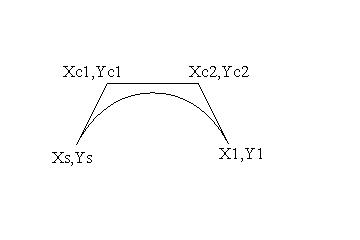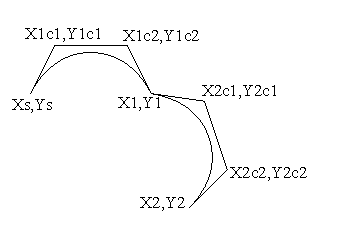Draw PolyBézier Curve

Purpose

The Draw PolyBézier Curve function draws a single Bézier curve or a series of connected Bézier curves.

Syntax

 Opcode format Opcode [ASCII] (Hex) Operand Format Comments Extended ASCII (Bezier ,[,,,]+[]) Absolute coordinates. Single-byte, binary operand [b] (62) [][]+ Relative coordinates.

Count The number of connected four-point Bézier curves to be drawn. In the binary operand case, a value of zero indicates that an extended count will follow. In the extended ASCII case, count may be any value larger than zero.

Ecount When count is zero, a 2-byte extended count follows allowing for PolyBézier curves of length 256 through 65,791, which are encoded as an integer (0 to 65,535).

Xs,Ys The position from which the 1st four-point Bézier curve starts from.

Xc1,Yc1 The first control point of the ith four-point Bézier curve.

Xc2,Yc2 The second control point of the ith four-point Bézier curve.

Xe,Ye The endpoint of the ith four-point Bézier curve. This point becomes the starting point for the (i + 1)th four-point Bézier curve, if there is one.

Details

A four-point Bézier curve is a smooth curve that passes through its two endpoints and is shaped by, but does not touch, its two control points. An example is shown in figure 1.Figure 1. Four-point Bézier curve

When the "Fill Mode" attribute is inactive (the default), the Draw PolyBézier function renders a curve based on the current line drawing attributes, which include "Color", "Visibility", "Line Weight", "Line Cap" and "Line Pattern."

When the "Fill Mode" attribute is active, the Draw PolyBézier function renders the interior of the region defined by the PolyBézier curve and the edge connecting Xs,Yx to the final Xe,Ye in a solid color. The color is based on the Polygon drawing attributes, which include "Color", and "Visibility."

Notes

In order to have a smooth joint when going from one four-point Bézier to the next in the series, the Xc2,Yc2 and the Xe,Ye of the previous Bézier must form a straight line with the Xc1,Yc1 point of the Bézier shown in figure 2.Figure 2. Smooth joint between two, four point Béziers

Implementation in WHIP! Versions

 Toolkit Function TBD Partial Full Revised in Draw PolyBézier Curve - - - -